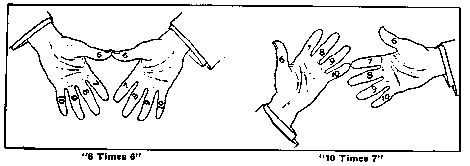All machinists use mathematics. Ask a machinist what would be the product of 9 times 8 and his ready reply would be 72, but change the figures a little and say 49 times 48 and the chances are that instead of replying at once he will have to figure it out with a pencil. By using the following method it is just as easy to tell at a glance what 99 times 99 are as 9 times 9. You will be able to multiply far beyond your most sanguine expectations.

In the first numbering, begin by holding your hands with the palms toward the body and make imaginary numbers on the thumbs and fingers as follows: Thumbs, 6; first fingers, 7; second fingers, 8; third fingers, 9, and fourth fingers, 10. Suppose you desire to multiply 8 by 9, put the eighth finger on one hand against the ninth finger of the other hand as shown."8 Times 9"

The two joined fingers and all the fingers above (calling the thumbs fingers) are called the upper fingers and each has a value of ten, which tens are added. All the fingers below the joined fingers are termed the lower fingers, and each of the lower fingers represents a unit value of one. The sum of the units on one hand should be multiplied by the sum of the units on the other hand. The total tens added to this last named sum will give the product desired. Thus: Referring to above picture or to your hands we find three tens on the left hand and four tens on the right, which would be 70. We also find two units on the left hand and one on the right. Two times one are two, and 70 plus 2 equals 72, or the product of 8 times 9.

Supposing 6 times 6 were the figures. Put your thumbs together; there are no fingers above, so the two thumbs represent two tens or 20; below the thumbs are four units on each hand, which would be 16, and 20 plus 16 equals 36, or the product of 6 times 6."6 Times 6" "10 Times 7"

Supposing 10 times 7 is desired. Put the little finger of the left hand against the first finger of the right hand. At a glance you see seven tens or 70. On the right hand you have three units and on the left nothing. Three times nothing gives you nothing and 70 plus nothing is 70.

In the second numbering, or numbers above 10, renumber your fingers; thumbs, 11; first fingers, 12, etc. Let us multiply 12 by 12.

Put together the tips of the fingers labeled 12. At a glance you see four tens or 40. At this point we leave the method explained in Case 1 and ignore the units (lower fingers) altogether. We go back to the upper fingers again"12 Times 12" and multiply the number of upper fingers used on the one hand by the number of upper fingers used on the other hand, viz., 2 times 2 equals 4. Adding 4 to 40 gives us 44. We now add 100 (because anything over 10 times 10 would make over 100) and we have 144, the product of 12 times 12.

The addition of 100 is arbitrary, but being simple it saves time and trouble. Still, if we wish, we might regard the four upper fingers in the above example as four twenties, or 80, and the six lower fingers as six tens, or 60; then returning to the upper fingers and multiplying the two on the right hand by the two on the left we would have 4; hence 80 plus 60 plus 4 equals 144; therefore the rule of adding the lump sum is much the quicker and easier method.

Above 10 times 10 the lump sum to add is 100; above 15 times 15 it is 200; above 20 times 20, 400; 25 times 25, 600, etc., etc., as high as you want to go.

In the third numbering to multiply above 15 renumber your fingers, beginning the thumbs with 16, first finger 17, and so on. Oppose the proper finger tips as before, the upper fingers representing a value of 20. Proceed as in the first numbering and add 200. Take For example 18 times 18.

At a glance we see six twenties plus 2 units on left hand times 2 units on right hand plus 200 equals 324.

In the fourth numbering the fingers are marked, thumbs, 21, first fingers 22, etc., the value of the upper fingers being 20. Proceed as in the second lumbering, adding 400 instead of 100."18 Times 18"

Above 25 times 25 the upper fingers represent a value of 30 each and after proceeding as in the third numbering you add 600 instead of 200.

This system can be carried as high as you want to go, but you must remember that for figures ending in 1, 2, 3, 4 and 5 proceed as in the second numbering. For figures ending in 6, 7, 8, 9 and 10 the third numbering applies.

Determine the value of the upper fingers whether they represent tens, twenties, thirties, forties, or what. For example, any two figures between 45 and 55, the value of the upper fingers would be 50, which is the half-way point between the two fives. In 82 times 84 the value of the upper fingers would be 80 (the half-way point between the two fives, 75 and 85, being 80). And the lump sum to add. Just three things to remember:

Which numbering is to follow, whether the one described in second or third numbering; the value which the upper fingers have; and, lastly, the lump sum to add, and you will be able to multiply faster and more accurately than you ever dreamed of before.